Check Full Chapter Explained - Continuity and Differentiability - Application of Derivatives (AOD) Class 12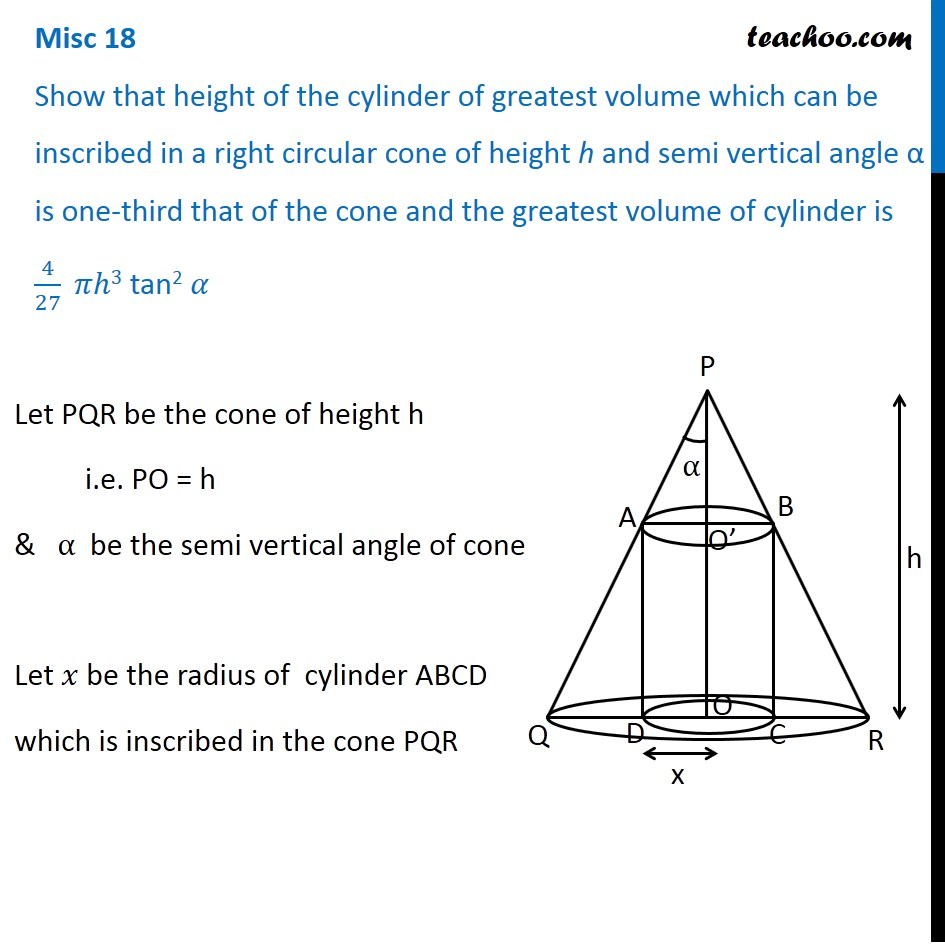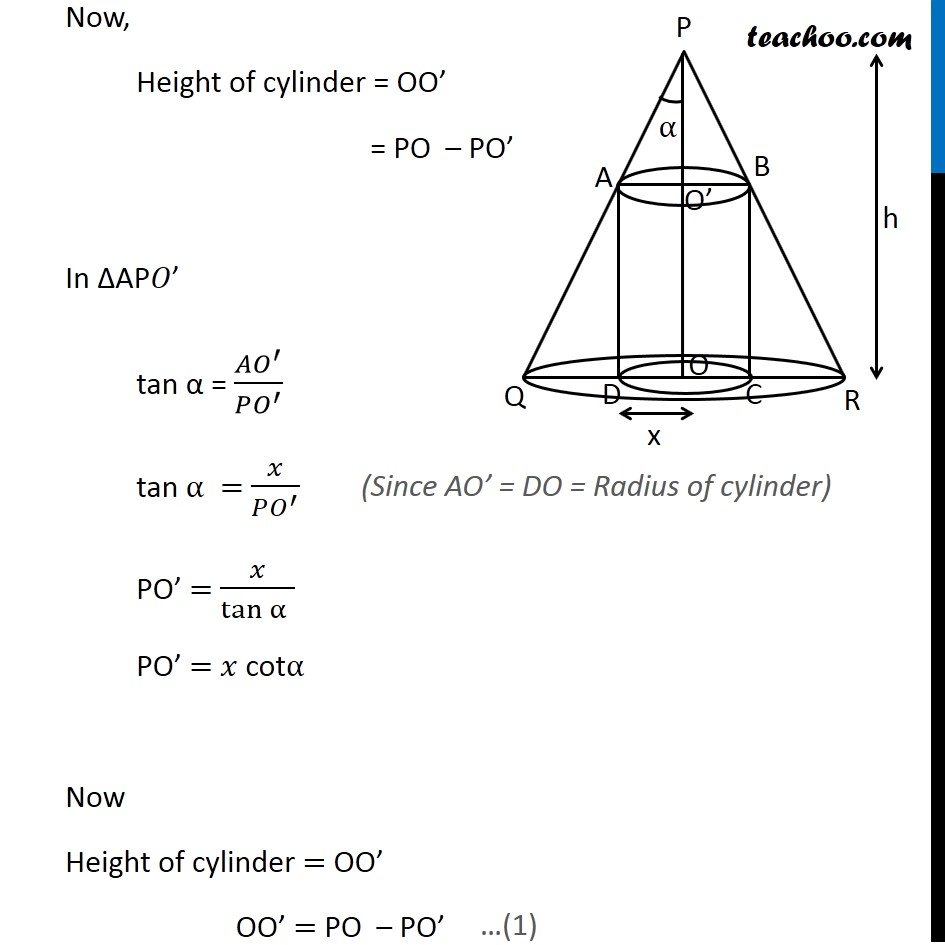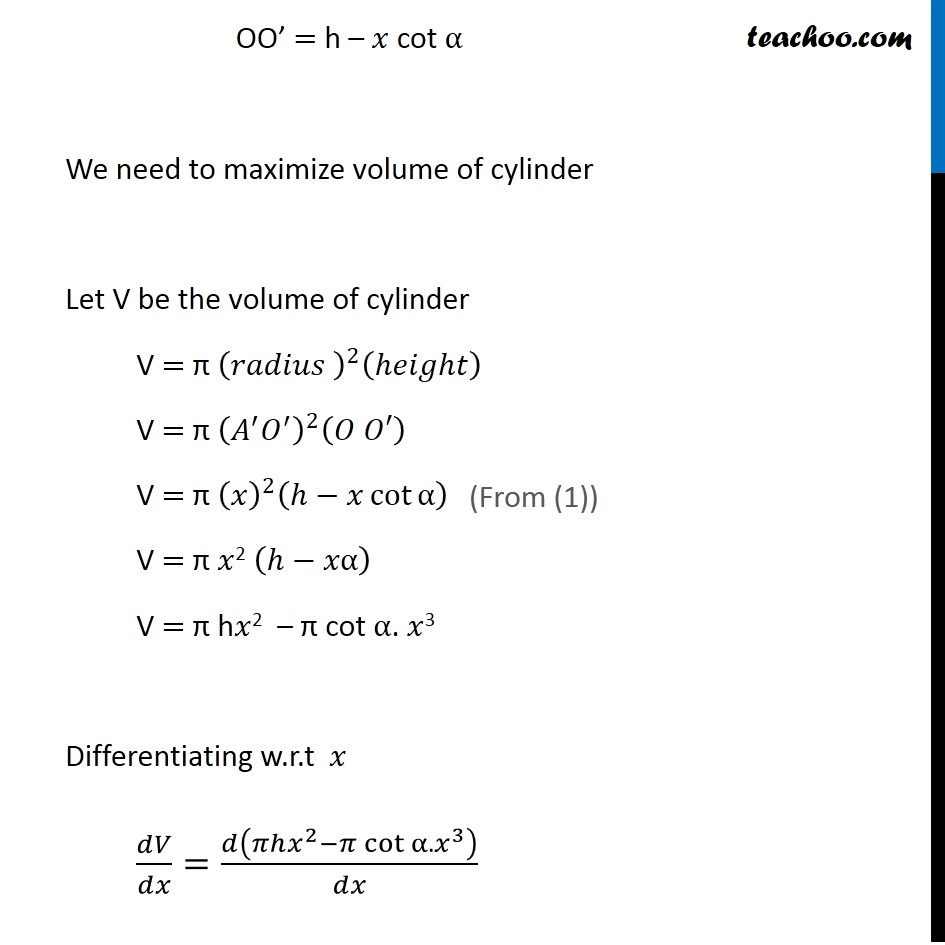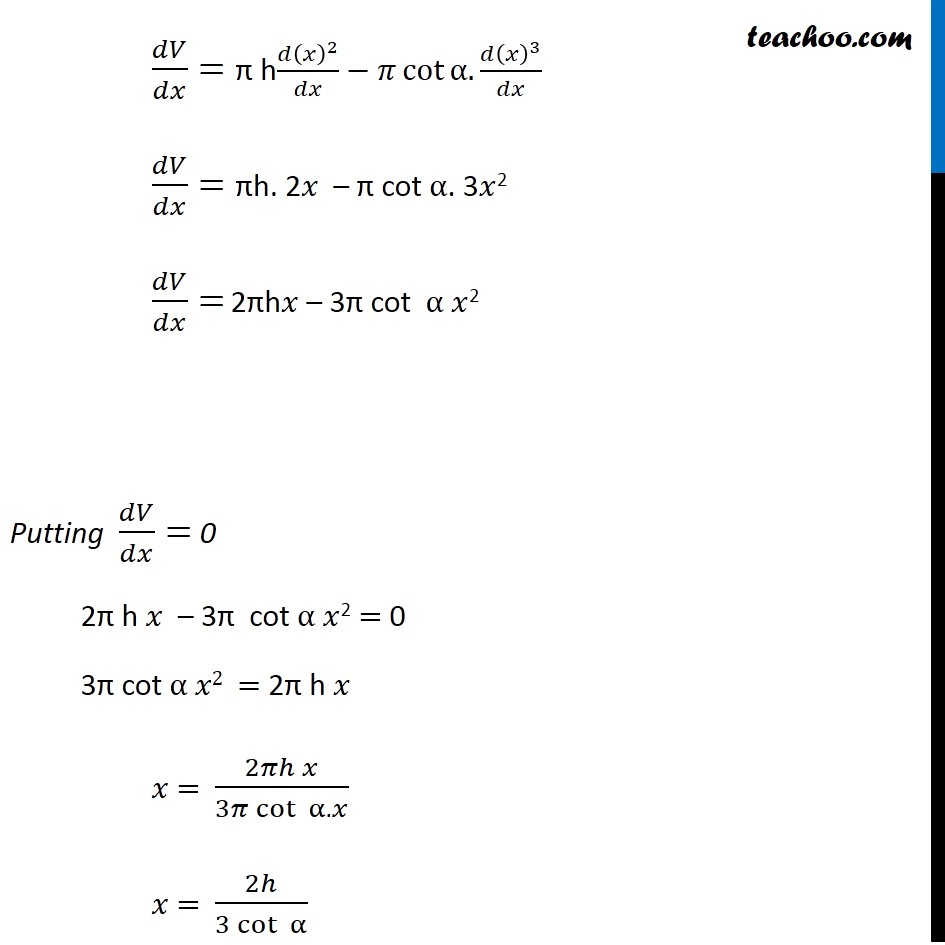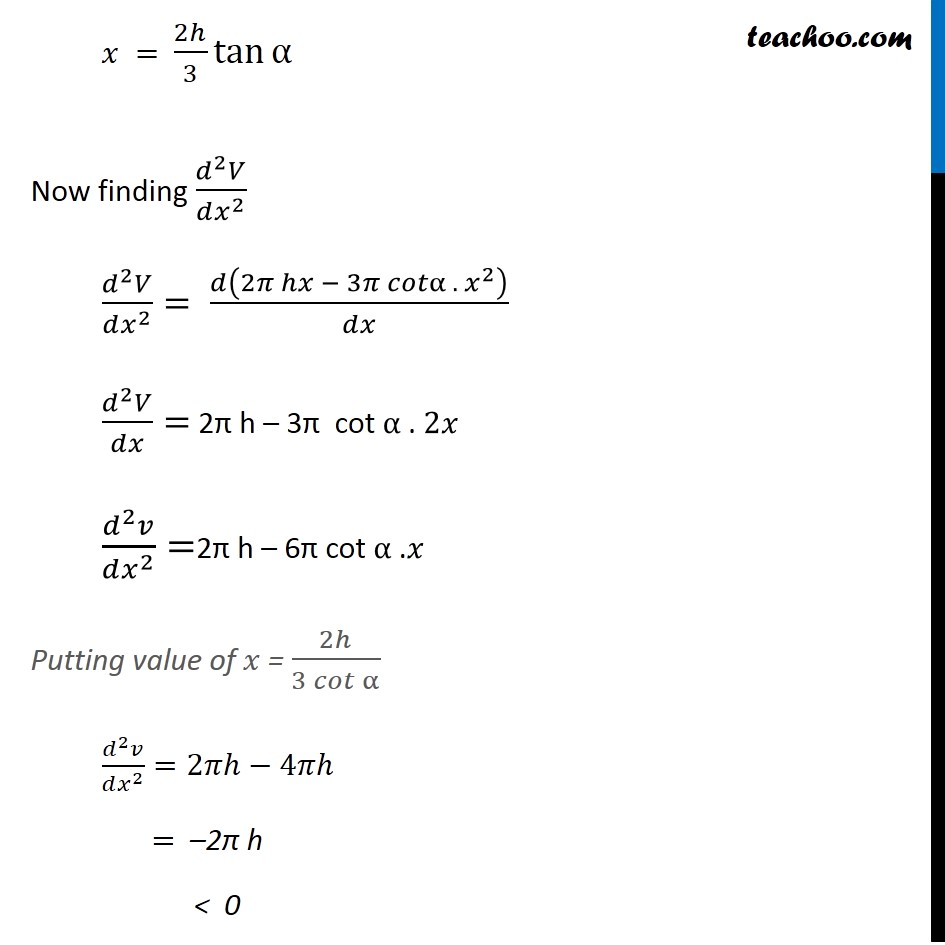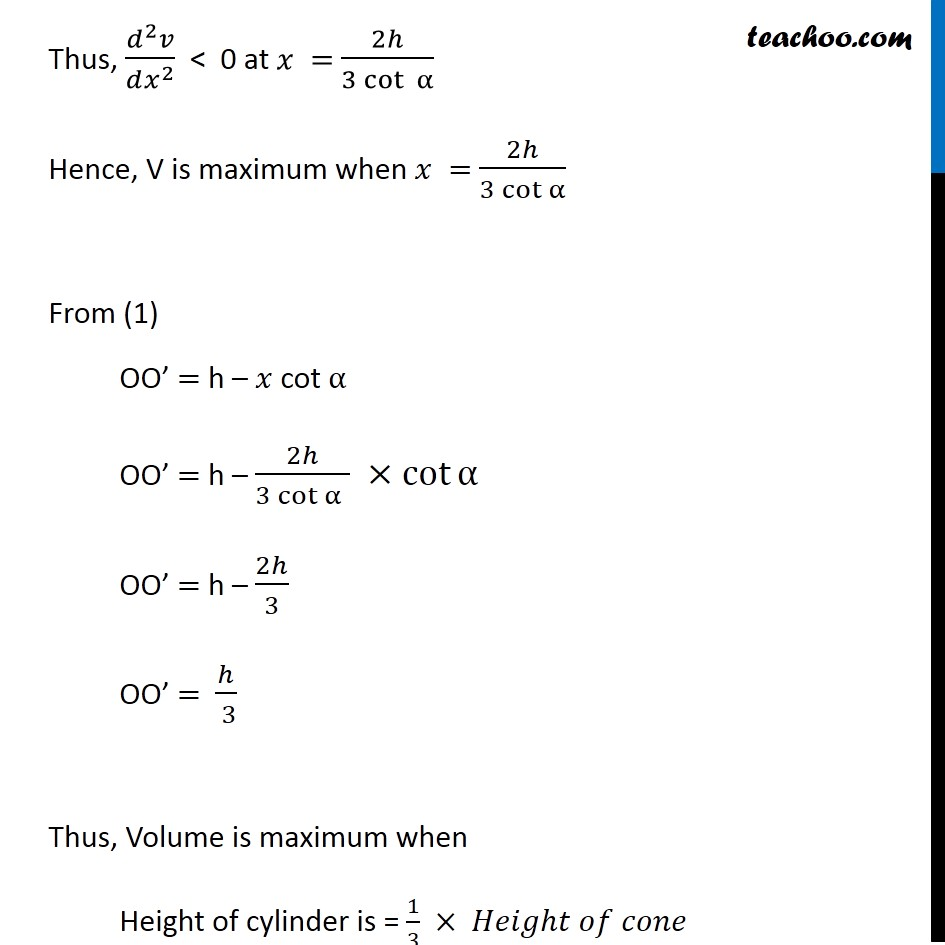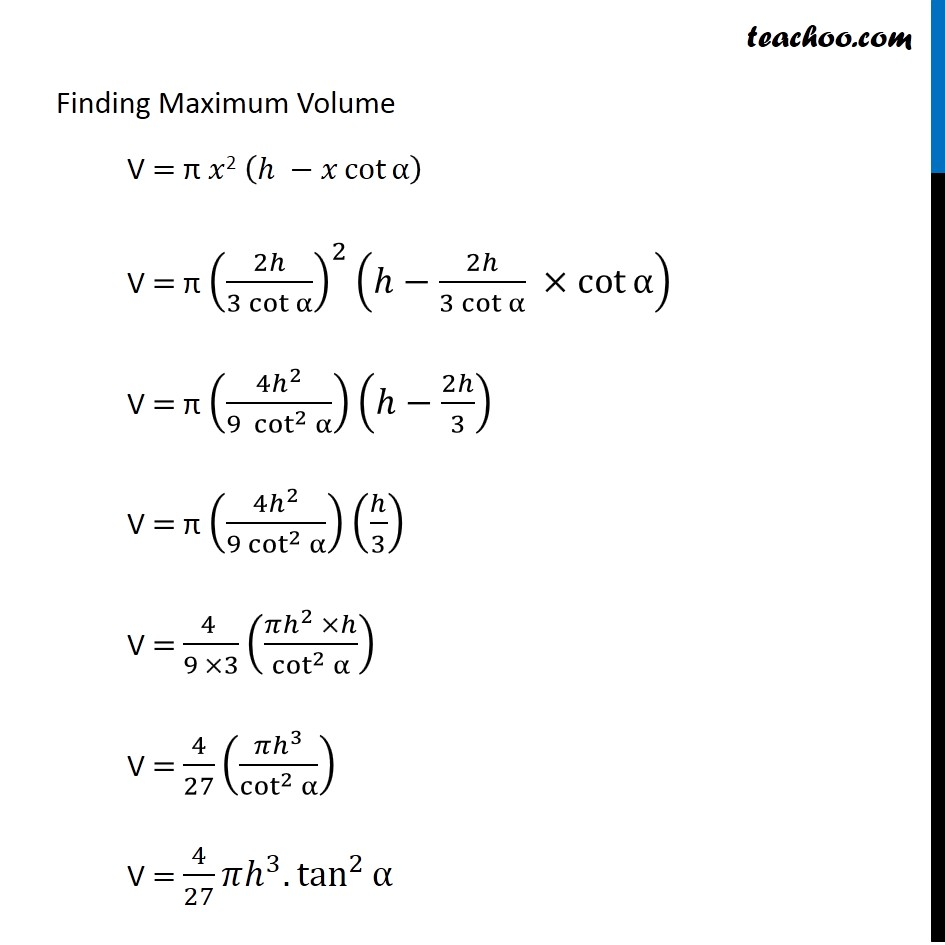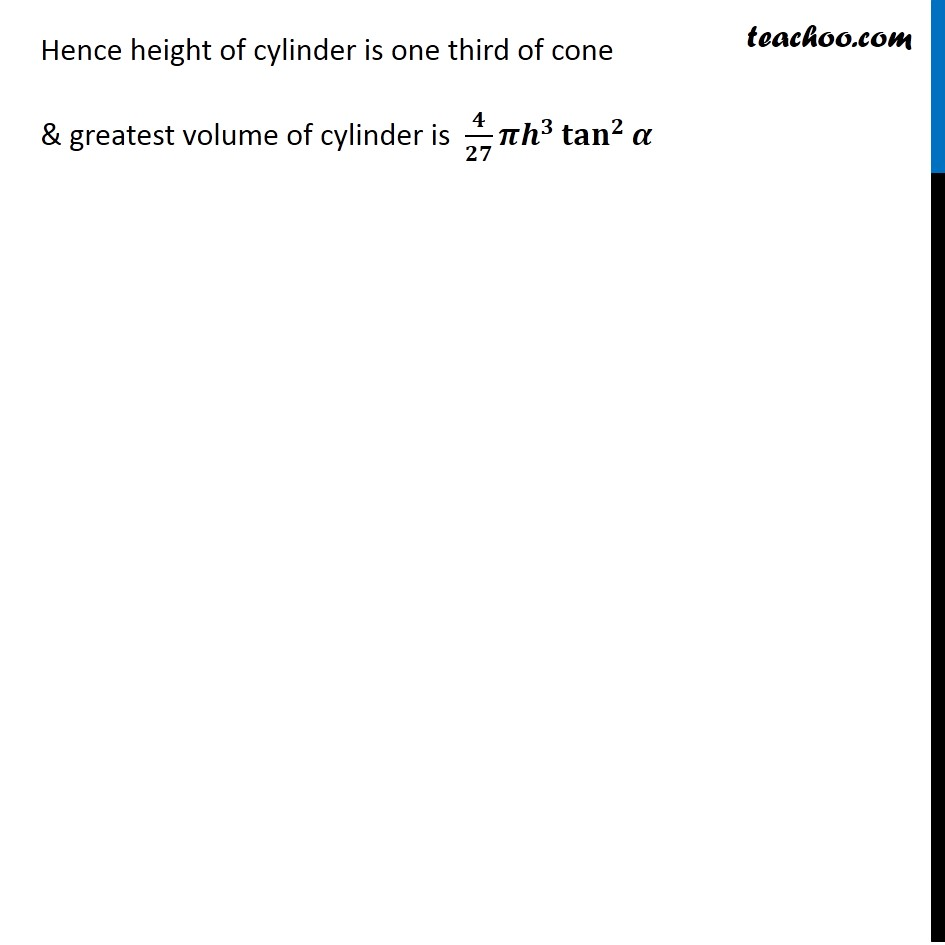1. Chapter 6 Class 12 Application of Derivatives
2. Serial order wise
3. Miscellaneous

Transcript

Misc 18 Show that height of the cylinder of greatest volume which can be inscribed in a right circular cone of height h and semi vertical angle α is one-third that of the cone and the greatest volume of cylinder is 4/27 𝜋ℎ3 tan2 𝛼 Let PQR be the cone of height h i.e. PO = h & α be the semi vertical angle of cone Let 𝑥 be the radius of cylinder ABCD which is inscribed in the cone PQR Now, Height of cylinder = OO’ = PO – PO’ In ∆AP𝑂’ tan α = (𝐴𝑂^′)/(𝑃𝑂^′ ) tan α = 𝑥/(𝑃𝑂^′ ) PO’ = 𝑥/tan⁡α" " PO’ = 𝑥 cotα Now Height of cylinder = OO’ OO’ = PO – PO’ (Since AO’ = DO = Radius of cylinder) OO’ = h – 𝑥 cot α We need to maximize volume of cylinder Let V be the volume of cylinder V = π (𝑟𝑎𝑑𝑖𝑢𝑠 )^2 (ℎ𝑒𝑖𝑔ℎ𝑡) V = π (𝐴^′ 𝑂^′ )^2 (𝑂 𝑂′) V = π (𝑥)^2 (ℎ−𝑥 cot⁡α ) V = π 𝑥2 (ℎ−𝑥α) V = π h𝑥2 – π cot α. 𝑥3 Differentiating w.r.t 𝑥 𝑑𝑉/𝑑𝑥=𝑑(𝜋ℎ𝑥^2−𝜋 cot⁡〖α.𝑥^3 〗 )/𝑑𝑥 (From (1)) 𝑑𝑉/𝑑𝑥= π h(𝑑(𝑥)^2)/𝑑𝑥−𝜋 cot⁡〖α.(𝑑(𝑥)^3)/𝑑𝑥〗 𝑑𝑉/𝑑𝑥= πh. 2𝑥 – π cot α. 3𝑥2 𝑑𝑉/𝑑𝑥= 2πh𝑥 – 3π cot α 𝑥2 Putting 𝑑𝑉/𝑑𝑥= 0 2π h 𝑥 – 3π cot α 𝑥2 = 0 3π cot α 𝑥2 = 2π h 𝑥 𝑥 = (2𝜋ℎ 𝑥)/(3𝜋 cot⁡〖 α.𝑥〗 ) 𝑥 = 2ℎ/(3 cot⁡〖 α〗 ) 𝑥 = 2ℎ/3 tan⁡α Now finding (𝑑^2 𝑉)/(𝑑𝑥^2 ) (𝑑^2 𝑉)/(𝑑𝑥^2 )= 𝑑(2𝜋 ℎ𝑥 − 3𝜋 𝑐𝑜𝑡α . 〖 𝑥〗^2 )/𝑑𝑥 (𝑑^2 𝑉)/𝑑𝑥= 2π h – 3π cot α . 2𝑥 (𝑑^2 𝑣)/(𝑑𝑥^2 )=2π h – 6π cot α . 𝑥 Putting value of 𝑥 = 2ℎ/(3 𝑐𝑜𝑡⁡α ) (𝑑^2 𝑣)/(𝑑𝑥^2 )=2𝜋ℎ−4𝜋ℎ = –2π h < 0 Thus, (𝑑^2 𝑣)/(𝑑𝑥^2 ) < 0 at 𝑥 = 2ℎ/(3 cot⁡〖 α〗 ) Hence, V is maximum when 𝑥 = 2ℎ/(3 cot⁡α ) From (1) OO’ = h – 𝑥 cot α OO’ = h – 2ℎ/(3 cot⁡α" " ) ×cot⁡α OO’ = h – 2ℎ/3 OO’ = ℎ/( 3) Thus, Volume is maximum when Height of cylinder is = 1/3 × 𝐻𝑒𝑖𝑔ℎ𝑡 𝑜𝑓 𝑐𝑜𝑛𝑒 Finding Maximum Volume V = π 𝑥2 (ℎ −𝑥 cot⁡α ) V = π (2ℎ/(3 cot⁡α ))^2 (ℎ−2ℎ/(3 cot⁡α ) ×cot⁡α ) V = π ((4ℎ^2)/(9 〖 cot〗^2⁡α ))(ℎ−2ℎ/3) V = π ((4ℎ^2)/(9 cot^2⁡α ))(ℎ/3) V = (4 )/(9 ×3) ((𝜋ℎ^2 ×ℎ)/cot^2⁡α ) V = 4/27 ((𝜋ℎ^3)/cot^2⁡α ) V = 4/27 𝜋ℎ^3.tan^2⁡α Hence height of cylinder is one third of cone & greatest volume of cylinder is 𝟒/𝟐𝟕 𝝅𝒉^𝟑 〖𝐭𝐚𝐧〗^𝟐⁡𝜶

Miscellaneous